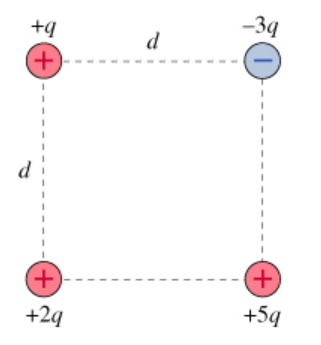# Problem: Four point charges form a square with sides of length d, as shown in the figure. In the questions that follow, use the constant k in place of 1/(4πϵ0).Part DWhat would be the kinetic energy K2q of charge 2q at a very large distance from the other charges?Express your answer in terms of q, d, and appropriate constants.Part EWhat will be the potential energy Utot of the system of charges when charge 2q is at a very large distance from the other charges?Express your answer in terms of q, d, and appropriate constants.

###### FREE Expert Solution

Potential energy between two charges is expressed as:

$\overline{){\mathbf{P}}{\mathbf{.}}{\mathbf{E}}{\mathbf{=}}\frac{\mathbf{k}{\mathbf{q}}_{\mathbf{1}}{\mathbf{q}}_{\mathbf{2}}}{\mathbf{r}}}$

Part D

When charge 2q has gotten a very large distance from the other charges, the final kinetic energy is equal to the initial potential energy.

The potential energy due to the charge 2q interacting with other charges is found as:

80% (254 ratings)###### Problem Details

Four point charges form a square with sides of length d, as shown in the figure. In the questions that follow, use the constant k in place of 1/(4πϵ0).Part D

What would be the kinetic energy K2q of charge 2q at a very large distance from the other charges?

Part E

What will be the potential energy Utot of the system of charges when charge 2q is at a very large distance from the other charges?